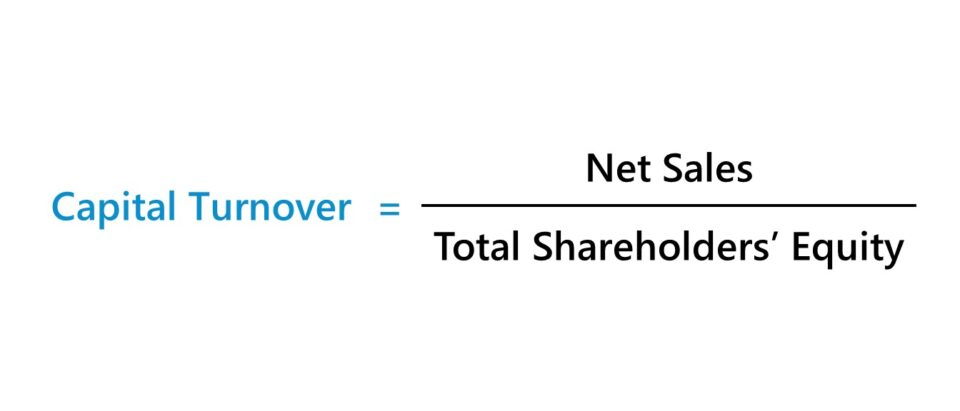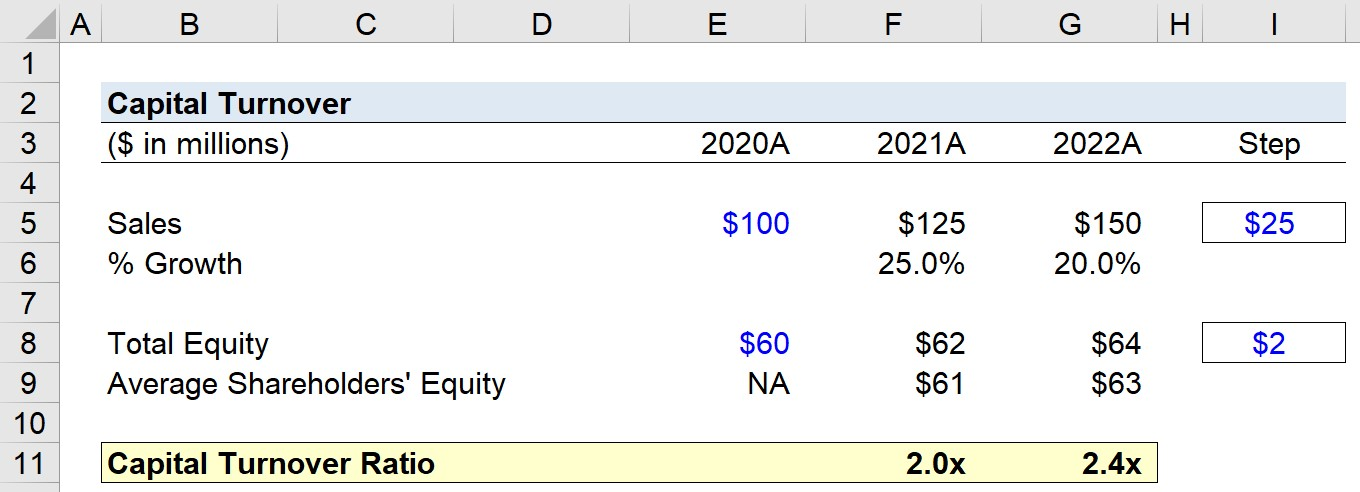Welcome to Wall Street Prep! Use code at checkout for 15% off.# Capital Turnover

Guide to Understanding Capital Turnover## How to Calculate Capital Turnover?

The capital turnover ratio estimates the operating efficiency of a company via its allocation of equity capital.

Expressed as a formula, capital turnover is the ratio between a company’s net sales and the average shareholders’ equity across a specified period.

The two components of the capital turnover ratio are: 1) net sales and 2) shareholders’ equity.

• Net Sales → The net sales of a company is the starting line item on the income statement that represents the total monetary value earned from the sale of goods and services in a specified period.
• Shareholders’ Equity → On the balance sheet, the shareholders’ equity section contains accounts like the owner’s equity (i.e. initial contribution), proceeds from the issuance of common stock or preferred stock, additional paid-in capital (APIC), and retained earnings.

Conceptually, the capital turnover therefore measures the proportion of a company’s sales generated per dollar of equity contribution.

The calculation of the capital turnover ratio is a three-step process:

1. Determine Net Sales in Specified Period
2. Calculate Average Shareholders’ Equity (i.e. Beginning of Period and End of Period Equity Balance Divided by Two)
3. Divide Net Sales by Average Shareholders’ Equity

## Capital Turnover Formula

The formula to calculate capital turnover is as follows.

Capital Turnover Ratio = Sales ÷ Average Shareholders’ Equity

Sales represent the “top line” of the income statement line, while inventory is found in the current assets section of the balance sheet.

Thus, there is a mismatch between the time period covered in the numerator and denominator.

• Income Statement → The income statement, or “profit and loss statement”, measures a company’s operating performance over a given period.
• Balance Sheet → The balance sheet portrays the value of a company’s assets, liabilities, and equity as of a specific date (i.e. “snapshot”).

The use of the average shareholders’ equity is an imperfect compromise to fix the mismatch in timing, yet it is a more accurate approach than simply using the ending balance.

Average Shareholders’ Equity = (Beginning Equity + Ending Equity) ÷ 2

However, the marginal improvement in accuracy is negligible in most cases, i.e. the insights derived, in all likelihood, will be nearly identical whether the average or ending balance is used in the formula.

## What is a Good Capital Turnover Ratio?

The capital turnover ratio is a method to understand a company’s operating efficiency, including analyze the upside in terms of its growth potential.

In general, a higher capital turnover ratio corresponds to greater upside in terms of revenue growth and profitability (and vice versa for lower ratios).

• Higher Capital Turnover → Increase in Revenue and Profits
• Lower Capital Turnover → Increase in Revenue and Profits

However, there are some pitfalls to the ratio that must be considered.

• Capital Intensity → The capital intensity of the industry the company operates in is a critical factor that impacts the capital turnover ratio. For instance, the turnover ratio tends to be much higher for service-oriented companies (e.g. consulting) than those with asset-heavy business models (e.g. manufacturing).
• Debt in Capital Structure (%) → The capital turnover ratio can also be artificially inflated, from the company relying more on debt financing to fund its operations and growth strategies, rather than equity financing. In such a case, the company is not necessarily more efficient at utilizing its equity, but instead adding more leverage to its capital structure introduces greater credit risk.

## Capital Turnover Calculator

We’ll now move to a modeling exercise, which you can access by filling out the form below.Submitting...

## Capital Turnover Calculation Example

Suppose we’re tasked with calculating the capital turnover ratio for a manufacturer with the following income statement and balance sheet data.

Selected Financial Data 2020A 2021A 2022A
Net Sales \$100 million \$125 million \$150 million
Total Shareholders’ Equity \$60 million \$62 million \$64 million

Starting off, we’ll determine the average shareholders’ equity balance for our historical periods.

• Average Shareholders’ Equity, 2020 to 2021 = \$61 million
• Average Shareholders’ Equity, 2021 to 2022 = \$63 million

The sales of a company over the course of the three-year historical period were provided as assumptions, i.e. \$100 million, \$125 million and \$150 million.

Our next step is to divide the sales from each period by the corresponding average shareholders’ equity balance to calculate the capital turnover.

Once completed, we arrive at a historical capital turnover ratio of 2.0x and 2.4x, which by itself, implies that the company is becoming more efficient over time at generating revenue per dollar of equity.

• Capital Turnover Ratio, 2020 to 2021 = 2.0x
• Capital Turnover Ratio, 2021 to 2022 = 2.4xStep-by-Step Online Course

### Everything You Need To Master Financial Modeling

Enroll in The Premium Package: Learn Financial Statement Modeling, DCF, M&A, LBO and Comps. The same training program used at top investment banks.